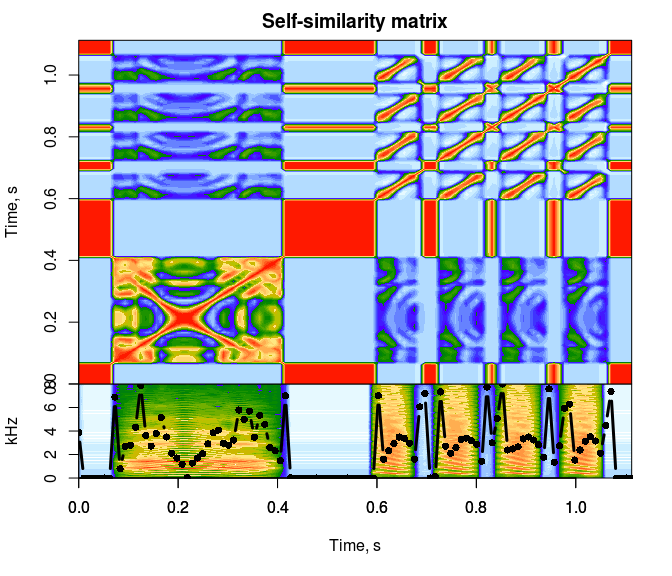# 1 Purpose

There are numerous programs out there for performing acoustic analysis, including several open-source options and R packages. For in-depth analysis of individual mammalian sounds it’s hard to beat PRAAT (batch processing is possible, but a bit tricky, because PRAAT uses its own, rather unusual scripting language). For bird sounds, a sophisticated tool is Sound Analysis Pro. In R, the most extensive acoustic toolkit by far is the seewave package. Soundgen builds upon the functionality of seewave, adding high-level functions for sound synthesis (see the vignette on sound synthesis) and acoustic analysis, particularly pitch tracking and audio segmentation.

Reasons to use `soundgen` for acoustic analysis might be:

1. User-friendly approach: a single call to the `analyzeFolder` function will give you a dataframe containing dozens of commonly used acoustic descriptors for each file in an entire folder. So if you’d rather get started with model-building without delving too deeply into acoustics, you are one line of code away from your dataset.
2. Flexible pitch tracking: soundgen uses several popular methods of pitch detection in parallel, followed by their integration and postprocessing. While the abundance of control parameters may initially seem daunting, for those who do wish to delve deeply this makes soundgen’s pitch tracker very versatile and offers a lot of power for high-precision analysis.
3. Audio segmentation with in-built optimization: the tools for syllable segmentation are again very flexible. Control parameters can even be optimized automatically, as long as you have a manually segmented training sample.

Many of the large variety of existing tools for acoustic analysis were designed with a particular type of sound in mind, usually human speech or bird songs. Soundgen’s pitch tracker was written to analyze human non-linguistic vocalizations like screams and laughs. These sounds are much harsher and noisier than ordinary speech and stand much closer to the vocalizations of other mammals than to human speech. In addition, the original corpus (Anikin & Persson, 2017) was collected from online videos, so that both sampling rate and microphone settings varied tremendously. From the very beginning, the focus has thus been on developing a pitch tracker and a segmenting tool that would be robust to noise and recording conditions. This makes soundgen highly suitable for performing acoustic analysis of animal vocalizations.

To summarize, you might want to look at soundgen’s tools for acoustic analysis if you are extracting a large number of acoustic predictors from a large number of audio files, for example:

• describing the vocal repertoire of a species (clustering),
• using machine learning for acoustic classification,
• comparing different classes of sounds in terms of specific acoustic predictors.

The most relevant functions are:

• `analyze`: analyzes a single sound and extracts a number of acoustic predictors such as pitch, harmonics-to-noise ratio, mean frequency, peak frequency, formants, etc. The output can be a summary per file, with each variable presented as mean / median / SD, or you can obtain detailed statistics per FFT frame.
• `analyzeFolder`: same as `analyze` but applied to all .wav files in a folder
• `segment`: finds syllables and bursts of energy in a single sound using its amplitude envelope
• `segmentFolder`: same as `segment` but applied to all .wav files in a folder
• `optimizePars`: optimizes control parameters of `segment` or `analyze` aiming to reproduce manual segmentation of a training sample
• `ssm`: produces a self-similarity matrix and calculates novelty as an alternative method of audio segmentation

TIP Soundgen’s functions for acoustic analysis are not meant to be exhaustive. MFCC extraction is readily available in R (e.g., with `tuneR::melfcc`), so there was no need to duplicate it in soundgen. Linear predictive coding (LPC) is also implemented in R (see `phonTools::lpc` and `phonTools::findformants`). As a convenience, `soundgen::analyze` shows the output of `phonTools::findformants`, but for serious formant analysis you might want to use an interactive program like PRAAT and check everything manually. A good approach may be to start with `soundgen::analyze` to get a table of many common acoustic predictors and then add some more using other R packages, software, or manual measurements. You can also customize `soundgen::analyze` to extract additional predictors.

This vignette is designed to show how soundgen can be used effectively to perform acoustic analysis. It assumes that the reader is already familiar with key concepts of phonetics and bioacoustics.

TIP This vignette mostly covers acoustic analysis with soundgen. In many cases, there are related R functions from other packages. For a tour-de-force overview of alternatives together with highly accessible theoretical explanations of sound characteristics, see Sueur (2018) “Sound analysis and synthesis with R”

# 2 Acoustic analysis with `analyze`

To demonstrate acoustic analysis in practice, let’s begin by generating a sound with a known pitch contour. To make pitch tracking less trivial and demonstrate some of its challenges, let’s add some noise, subharmonics, and jitter:

``````library(soundgen)
s1 = soundgen(sylLen = 900, temperature = 0,
pitch = list(time = c(0, .3, .8, 1),
value = c(300, 900, 400, 2300)),
noise = c(-40, 0), subDep = 100,
jitterDep = 0.5, nonlinBalance = 100,
plot = TRUE, ylim = c(0, 4))``````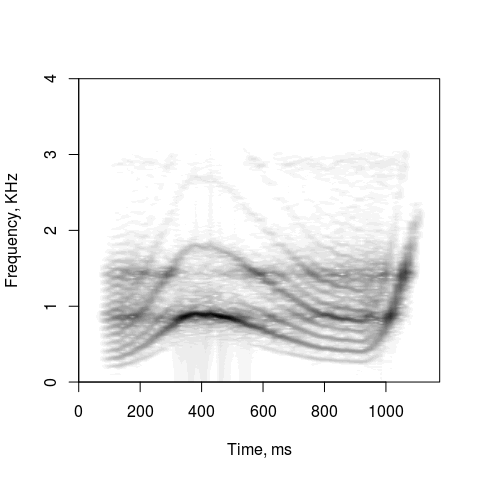``# playme(s1)  # replay as many times as needed w/o re-synthesizing the sound``

The contour of F0 is determined by our pitch anchors, so we can calculate the true median pitch:

``````true_pitch = getSmoothContour(anchors = list(time = c(0, .3, .8, 1),
value = c(300, 900, 400, 2300)),
len = 1000)  # any length will do
median(true_pitch)  # 611 Hz``````
``##  611.1947``

## 2.1 Basic principles

At the heart of acoustic analysis with soundgen is the short-time Fourier transform (STFT): we look at one short segment of sound at a time (one FFT frame), analyze its spectrum using Fast Fourier Transform (FFT), and then move on to the next - perhaps overlapping - frame. As the analysis window slides along the signal, STFT shows which frequencies it contains at different points of time. The nuts and bolts of STFT are beyond the scope of this vignette, but they can be found in just about any textbook on phonetics, acoustics, digital signal processing, etc. For a quick R-friendly introduction, see seewavevignette on acoustic analysis.

Putting the spectra of all frames together, we get a spectrogram. `analyze` calls another function from soundgen package, `spectrogram`, to produce a spectrogram and then plot pitch candidates on top of it. `spectrogram` is a modified version of `seewave::spectro` with added routines for removing background noise, controlling contrast and brightness, adding median smoothing, etc (see the examples in `?spectrogram`). To analyze a sound with default settings and plot its spectrogram, all we need to specify is its sampling rate (the default in soundgen is 16000 Hz):

``````a1 = analyze(s1, samplingRate = 16000, plot = TRUE, ylim = c(0, 4))
# summary(a1)  # many acoustic predictors measured for each FFT frame
median(true_pitch)  # true value, as synthesized above``````
``##  611.1947``
``median(a1\$pitch, na.rm = TRUE)  # our estimate``
``##  566.4659``
``````# Pitch postprocessing is stochastic (see below), so the contour may vary.
# Many candidates are off target, mainly b/c of misleading subharmonics.``````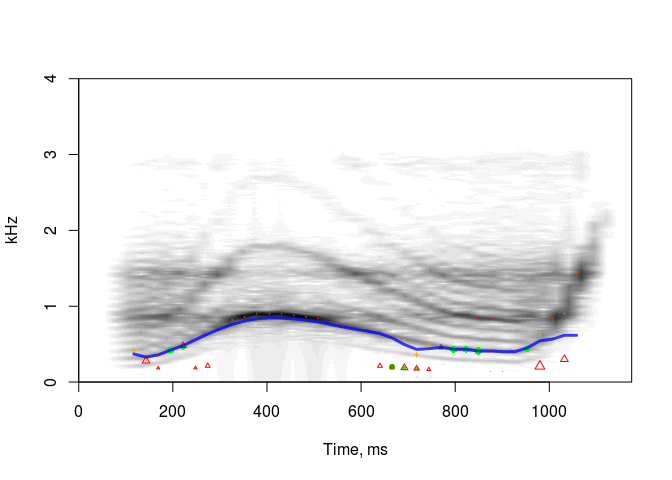There are several key parameters that control the behavior of STFT and affect all extracted acoustic variables. The same parameters serve as arguments to `spectrogram`. As a result, you can immediately see what frame-by-frame input you have fed into the algorithm for acoustic analysis by visually inspecting the produced spectrogram. If you can hear F0, but can’t see individual harmonics in the spectrogram, the pitch tracker probably will not see them, either, and will therefore fail to detect F0 correctly. The first remedy is thus to adjust STFT settings, using the spectrogram for visual feedback:

• `windowLength`: the length of sliding STFT window. Longer windows (e.g., 40 - 50 ms) improve frequency resolution at the expense of time resolution, so they are good for detecting relatively low, slowly changing F0, as in human moans or grunts. Shorter windows (e.g., 5 - 10 ms) improve time resolution at the expense of frequency resolution, so they are good for visualizing formants or tracking high-frequency, rapidly changing F0 as in bird chirps or dolphin whistles.
• `step`: the step of sliding STFT window. For example, if `windowLength = 50` and `step = 25`, each time we move the analysis frame, there is a 50% overlap with the previous frame. This introduces redundancy into the analysis, but it also - to some limited extent - improves time resolution while maintaining relatively high frequency resolution. The main cost of small steps (large overlap) is processing time, but very large overlap is not always desirable, even when processing time is not an issue. If some audio segments are problematic (e.g., very noisy), pitch contour may actually be more accurate with relatively large steps and more smoothing. It is therefore best to check the results with different steps and/or run formal optimization (remember to adjust smoothing and other postprocessing parameters together with STFT settings).
• `wn`: the type of windowing function used to taper the analysis frame during STFT. In practice the windowing function doesn’t seem to have a major effect on the result, as long as you choose something reasonable like gaussian, hanning, or bartlett.
• `zp`: zero-padding. You can use a short STFT window and improve its frequency resolution by padding each frame with zeroes. This is a computational trick that - again, to some limited extent - improves frequency resolution while maintaining relatively high time resolution.
• `silence`: frames with amplitude below silence threshold are not analyzed at all. Quiet frames are harder to analyze, because their signal-to-noise ratio is lower. As a result, we want to strike a good balance. Setting `silence` too low (close to 0) produces a lot of garbage, as the algorithm tries to analyze frames that are essentially just background noise without any signal. Setting `silence` too high (close to 1) excludes too many perfectly good frames, misrepresenting the signal. In soundgen `silence` is dynamically updated: it can never be lower than specified, but it may be raised to the minimum root mean square amplitude of all frames, if this minimum is higher than `silence`. This ensures that empty frames are not analyzed in recordings with unusually high levels of steady background noise (e.g., microphone hiss).

## 2.2 Basic spectral descriptives

Apart from pitch tracking, `analyze` calculates and returns several acoustic characteristics from each non-silent FFT frame:

• `time`: time of the middle of each frame (ms)
• `ampl`: root mean square of amplitude per frame, calculated as `sqrt(mean(frame ^ 2))`
• `amplVoiced`: the same as `ampl` for voiced frames and `NA` for unvoiced frames
• `dom`: lowest dominant frequency band (Hz) (see “Pitch tracking methods / Dominant frequency”)
• `entropy`: Weiner entropy of the spectrum of the current frame. Close to 0: pure tone or tonal sound with nearly all energy in harmonics; close to 1: white noise
• `f1_freq`, `f1_width`, …: the frequency and bandwidth of the first `nFormants` formants per FFT frame, as calculated by `phonTools::findformants` with default settings
• `harmonics`: the amount of energy in upper harmonics, namely the ratio of total spectral power above 1.25 x F0 to the total spectral power below 1.25 x F0 (dB)
• `HNR`: harmonics-to-noise ratio (dB), a measure of harmonicity returned by `soundgen:::getPitchAutocor()` (see “Pitch tracking methods / Autocorrelation”). If HNR = 0 dB, there is as much energy in harmonics as in noise
• `medianFreq`: 50th quantile of the frame’s spectrum
• `peakFreq`: the frequency with maximum spectral power (Hz)
• `peakFreqCut`: the frequency with maximum spectral power below `cutFreq` (Hz)
• `quartile25`, `quartile50`, `quartile75`: the 25th, 50th, and 75th quantiles of the spectrum below `cutFreq` (Hz)
• `specCentroid`: the center of gravity of the frame’s spectrum, first spectral moment (Hz)
• `specCentroidCut`: the center of gravity of the frame’s spectrum below cutFreq
• `specSlope`: the slope of linear regression fit to the spectrum below `cutFreq`
• `voiced`: is the current FFT frame voiced? TRUE / FALSE

## 2.3 Custom spectral descriptives

The function `soundgen::analyze` returns a few spectral descriptives that make sense for nonverbal vocalizations, but additional predictors may be useful for other applications (bird songs, non-biological sounds, etc.). One way to obtain extra predictors is to add the necessary code to the internal function `soundgen:::analyzeFrame()` and to `soundgen::analyze()`. If you want deltas, they can be extracted directly from the output of `analyze(..., summary = FALSE)`. But in many cases the easiest solution may be to just extract the spectra and then process them manually, without calling `analyze()`. In fact, many popular spectral descriptors are mathematically trivial to derive - all you need is the power spectrum for each STFT frame, or perhaps even the average spectrum of the entire sound. Here is how you can get these spectra.

For the average spectrum of an entire sound, go no further than `seewave::spec` or `seewave::meanspec`:

``````spec = seewave::spec(s1, f = 16000, plot = FALSE)  # FFT of the entire sound
avSpec = seewave::meanspec(s1, f = 16000, plot = FALSE)  # STFT followed by averaging
# either way, you get a dataframe with two columns: frequencies and their power
``````##            x            y
## [1,] 0.00000 0.0000893304
## [2,] 0.03125 0.0001254940
## [3,] 0.06250 0.0002629925
## [4,] 0.09375 0.0004060052
## [5,] 0.12500 0.0004356378
## [6,] 0.15625 0.0007267332``````

If you are interested in how the spectrum changes over time, extract frame-by-frame spectra - for example, with `spectrogram(..., output = 'original')`:

``````spgm = spectrogram(s1, samplingRate = 16000, output = 'original', plot = FALSE)
# rownames give you frequencies, colnames are time stamps
str(spgm)``````
``````##  num [1:400, 1:75] 3.96e-04 1.81e-04 1.17e-05 2.29e-06 1.30e-06 ...
##  - attr(*, "dimnames")=List of 2
##   ..\$ : chr [1:400] "0" "0.02" "0.04" "0.06" ...
##   ..\$ : chr [1:75] "0" "15.8783783783784" "31.7567567567568" "47.6351351351351" ...``````

Let’s say you are working with frame-by-frame spectra and want to calculate skewness, the 66.6th percentile, and the ratio of energy above/below 500 Hz. Before you go hunting for a piece of software that returns exactly those descriptors, consider this. Once you have normalized the power spectrum to add up to 1, it basically becomes a probability density function (pdf), so you can summarize it in the same way as you would any other distribution of a random variable. Look up the formulas you need and just do the raw math:

``````# Transform power to pdf (all columns should sum to 1):
spgm_norm = apply(spgm, 2, function(x) x / sum(x))
# Set up a dataframe to store the output
out = data.frame(skew = rep(NA, ncol(spgm)),
quantile66 = NA,
ratio500 = NA)
# Process each STFT frame
for (i in 1:ncol(spgm_norm)) {
# Power spectrum for this frame
df = data.frame(
freq = as.numeric(rownames(spgm_norm)),  # frequency (kHz)
d = spgm_norm[, i]                       # density
)
# plot(df, type = 'l')

# Skewness (see https://en.wikipedia.org/wiki/Central_moment)
m = sum(df\$freq * df\$d)  # spectral centroid, kHz
out\$skew[i] = sum((df\$freq - m)^3 * df\$d)

# 66.6th percentile (2/3 of density below this frequency)
out\$quantile66[i] = df\$freq[min(which(cumsum(df\$d) >= 2/3))]  # in kHz

# Energy above/below 500 Hz
out\$ratio500[i] = sum(df\$d[df\$freq >= .5]) / sum(df\$d[df\$freq < .5])
}
summary(out)``````
``````##       skew           quantile66       ratio500
##  Min.   :0.04029   Min.   :0.020   Min.   :  0.0016
##  1st Qu.:0.23385   1st Qu.:0.900   1st Qu.: 11.5416
##  Median :0.57526   Median :1.080   Median : 47.9299
##  Mean   :0.81609   Mean   :1.049   Mean   : 92.4295
##  3rd Qu.:1.08085   3rd Qu.:1.230   3rd Qu.:120.5343
##  Max.   :4.42959   Max.   :2.360   Max.   :827.9901``````

If you need to do this analysis repeatedly, just wrap the code into your own function that takes a wav file as input and returns all these spectral descriptives. You can also save the actual spectra of different sound files and add them up to obtain an average spectrum across multiple sound files, work with cochleograms instead of power spectra (check out `tuneR::melfcc`), etc. Be your own boss!

## 2.4 Pitch tracking

If you look at the source code of `soundgen::analyze()` and embedded functions, you will see that almost all of this code deals with a single acoustic characteristic: fundamental frequency (F0) or its perceptual equivalent, pitch. That’s because pitch is both highly salient to listeners and notoriously difficult to measure accurately. The approach followed with soundgen’s pitch tracker is to use several different estimates of F0, each of which is better suited to certain types of sounds. You can use any pitch tracker individually, but their output is also automatically integrated and postprocessed so as to generate the best overall estimate of frame-by-frame pitch. There are four currently implemented classes of pitch estimates in soundgen: autocorrelation, lowest dominant frequency, cepstrum, and spectrum (ratios of harmonics). These four methods of pitch estimation are not treated as completely independent in soundgen. Autocorrelation is performed first to provide an initial guess at the likely pitch and harmonics-to-noise ratio (HNR) of an FFT frame, and then this information is used to adjust the expectations of the cepstral and spectral algorithms. In particular, if autocorrelation suggests that the pitch is high, confidence in cepstral estimates is attenuated; and if autocorrelation suggests that HNR is low, thresholds for spectral peak detection are raised, making spectral pitch estimates more conservative.

The plot below shows a spectrogram of the sound with overlaid pitch candidates: yellow circles = autocorrelation pitch estimate (`pitchAutocor`), red triangles = spectral pitch estimate (`pitchSpec`), orange crosses = lowest dominant frequency band (`dom`). The size of each point shows the certainty of estimation: smaller points are calculated with lower certainty and have less weight when all candidates are integrated into the final pitch contour (blue line). To specify which pitch tracking methods should be employed, use `pitchMethods`. For example, we can also include cepstral estimates (violet envelopes):

``````a = analyze(s1, samplingRate = 16000, plot = TRUE, ylim = c(0, 4),
pitchMethods = c('autocor', 'cep', 'dom', 'spec'))``````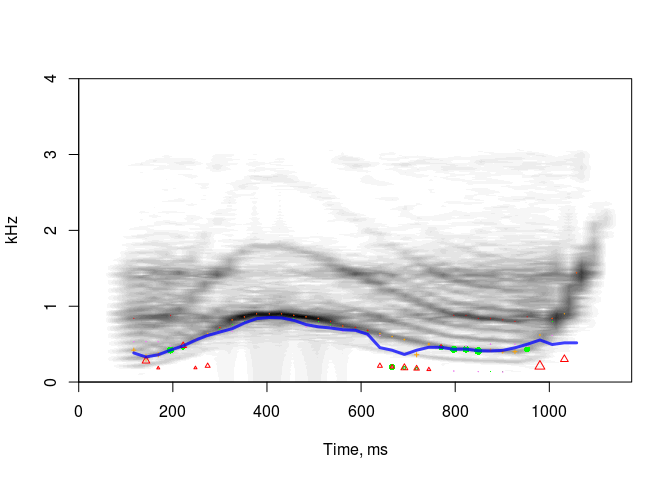### 2.4.1 General settings

`analyze` has a few arguments that affect all methods of pitch tracking:

• `entropyThres`: all non-silent frames are analyzed to produce basic spectral descriptives. However, pitch tracking is both computationally costly and can be misleading if applied to obviously voiceless frames. To define what an “obviously voiceless” frame is, we set some cutoff value of Weiner entropy, above which we don’t want to even try pitch tracking. To disable this feature and track pitch in all non-silent frames, set `entropyThres` to 1.
• `pitchFloor`, `pitchCeiling`: absolute thresholds for pitch candidates. No values outside these bounds will be considered.
• `priorMean` and `priorSD` specify the mean and sd of gamma distribution describing our prior knowledge about the most likely pitch values. The prior works by scaling the certainties associated with particular pitch candidates: candidates more than a couple of `priorSD`’s from `priorMean` have their weight reduced to nearly zero. Based on (limited) testing, the mildly informative default prior in `analyze` does improve the accuracy of pitch tracking in human vocalizations. Prior values are specified in semitones above C-5 (~0.5 Hz), and densities are calculated on a musical scale. For example, if we expect F0 values of about 300 Hz plus-minus half an octave (6 semitones), a prior can be defined as `priorMean = HzToSemitones(300), priorSD = 6`. For convenience, the prior can be plotted directly from `analyze`:
``````par(mfrow = c(1, 2))
# default prior in soundgen
a1 = analyze(s1, samplingRate = 16000, plot = FALSE, priorPlot = TRUE,
priorMean = HzToSemitones(300), priorSD = 6)
# narrow peak at 2 kHz
a2 = analyze(s1, samplingRate = 16000, plot = FALSE, priorPlot = TRUE,
priorMean = HzToSemitones(2000), priorSD = 1)
par(mfrow = c(1, 1))``````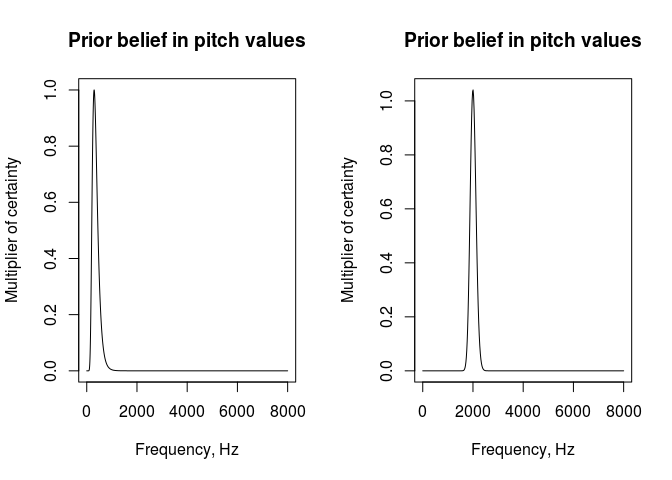TIP The final pitch contour can still pass through low-certainty candidates, especially if no better candidates are available. You can therefore think of the prior as a soft alternative (or addition) to the inflexible bounds of `pitchFloor` and `pitchCeiling`

• `nCands`: maximum number of pitch candidates to use per method. This only affects `pitchAutocor`, `pitchCep`, and `pitchSpec`. `dom` never returns more than one candidate per frame, since it doesn’t make sense to consider several lowest dominant frequency bands - this simply drags the final pitch contour upwards without improving the accuracy.
• `minVoicedCands`: minimum number of pitch candidates that have to be defined to consider a frame voiced. It defaults to ‘autom’, which means 2 if `dom` is among the candidates and 1 otherwise. The reason is that `dom` is usually defined, even if the frame is clearly voiceless, so we want another pitch candidate in addition to `dom` before we classify the frame as voiced.

### 2.4.2 Pitch tracking methods

Having looked at the general settings, it is time to consider the theoretical principles behind each pitch tracking method, together with arguments to `analyze` that can be used to tweak each one.

#### 2.4.2.1 Autocorrelation

Time domain: pitch by autocorrelation, PRAAT, `pitchAutocor`.

This is an R implementation of the algorithm used in the popular open-source program PRAAT (Boersma, 1993). The basic idea is that a harmonic signal correlates with itself most strongly at a delay equal to the period of its fundamental frequency (F0). Peaks in the autocorrelation function are thus treated as potential pitch candidates. The main trick is to choose an appropriate windowing function and adjust for its own autocorrelation. Compared to other methods implemented in soundgen, pitch estimates based on autocorrelation appear to be particularly accurate for relatively high values of F0. The settings that control `pitchAutocor` are:

• `autocorThres`: voicing threshold, defaults to 0.7. This means that peaks in the autocorrelation function have to be at least 0.7 in height (1 = perfect autocorrelation). A lower threshold produces more false positives (F0 is detected in voiceless, noisy frames), whereas a higher threshold produces more accurate values F0 at the expense of failing to detect F0 in noisier frames.
• `autocorSmooth`: the width of smoothing interval (in bins) for finding peaks in the autocorrelation function. If left NULL, it defaults to 7 for sampling rate 44100 and smaller odd numbers for lower sampling rate.

To use only autocorrelation pitch tracking, but with lower-than-default voicing threshold and more candidates, we can do something like this (prior is disabled so as not to influence the certainties of different pitch candidates):

``````a = analyze(s1, samplingRate = 16000,
plot = TRUE, ylim = c(0, 4), priorMean = NA,
pitchMethods = 'autocor',
autocorThres = .45,
nCands = 3)``````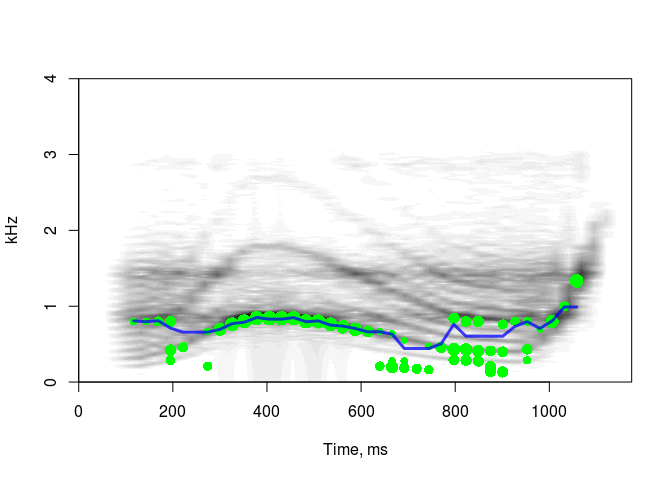#### 2.4.2.2 Dominant frequency

Frequency domain: the lowest dominant frequency band, `dom`.

If the sound is harmonic and relatively noise-free, the spectrum of a frame typically has little energy below F0. It is therefore likely that the first sizable peak in the spectrum is in fact F0, and all we have to do is choose a reasonable threshold. Naturally, there are cases of missing F0 and misleading low-frequency noises. Nevertheless, this simple estimate is often surprisingly accurate, and it may be our best shot when the vocal cords are vibrating in a chaotic fashion (deterministic chaos). For example, sounds such as roars lack clear harmonics but are perceived as voiced, and the lowest dominant frequency band often corresponds to perceived pitch.

The settings that control `dom` are:

• `domThres` (defaults to 0.1, range 0 to 1): to find the lowest dominant frequency band, we look for the lowest frequency with amplitude at least `domThres`. This key setting has to be high enough to exclude accidental low-frequency noises, but low enough not to miss F0. As a result, the optimal level depends a lot on the type of sound analyzed and recording conditions.
• `domSmooth` (defaults to 220 Hz): the width of smoothing interval (Hz) for finding the lowest spectral peak. The idea is that we are less likely to hit upon some accidental spectral noise and find the lowest harmonic (or the lowest spectral band with significant power) if we apply some smoothing to the spectrum of an FFT frame, in this case a moving median.

For the sound we are trying to analyze, we can increase `domSmooth` and/or raise `domThres` to ignore the subharmonics and trace the true pitch contour:

``````a = analyze(s1,
samplingRate = 16000, plot = TRUE, ylim = c(0, 4), priorMean = NA,
pitchMethods = 'dom',
domThres = .1,
domSmooth = 500)``````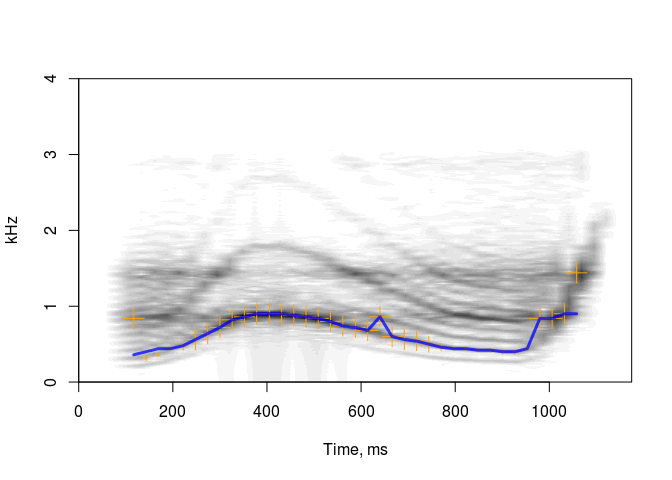#### 2.4.2.3 Cepstrum

Frequency domain: pitch by cepstrum, `pitchCep`.

Cepstrum is the FFT of log-spectrum. It may be a bit challenging to wrap one’s head around, but the main idea is quite simple: just as FFT is a way to find periodicity in a signal, cepstrum is a way to find periodicity in the spectrum. In other words, if the spectrum contains regularly spaced harmonics, its FFT will contain a peak corresponding to this regularity. And since the distance between harmonics equals the fundamental frequency, this cepstral peak gives us F0. Actually, in soundgen the FFT is applied to raw spectrum, not log-spectrum, since it appears to produce better results. Cepstrum is not very useful when F0 is so high that the spectrum contains only a few harmonics, so soundgen automatically discounts the contribution of high-frequency cepstral estimates. Cepstral pitch tracking is disabled by default, since this method is both slower and less robust for human non-linguistic vocalizations. Depending on the type of analyzed audio, however, both the accuracy of different pitch tracking methods and their optimal parameters may change (see the section on optimization below).

The settings that control `pitchCep` are:

• `cepThres`: voicing threshold (defaults to 0.3).
• `cepSmooth`: the width of smoothing interval (in bins) for finding peaks in the cepstrum. If left NULL, it defaults to 31 for sampling rate 44100 and smaller odd numbers for lower values of sampling rate.
• `cepZp` (defaults to 0): zero-padding of the spectrum used for cepstral pitch detection (points). Zero-padding may improve the precision of cepstral pitch detection, but it also slows down the algorithm.
``````a = analyze(s1,
samplingRate = 16000, plot = TRUE, ylim = c(0, 4), priorMean = NA,
pitchMethods = 'cep',
cepThres = .3,
cepSmooth = 3,
nCands = 2)``````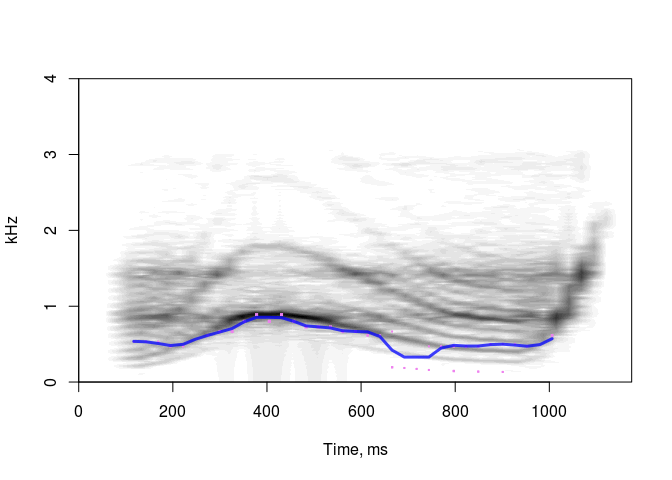#### 2.4.2.4 Spectrum

Frequency domain: ratios of harmonics, BaNa, `pitchSpec`.

All harmonics are multiples of the fundamental frequency. The ratio of two neighboring harmonics is thus predictably related to their rank relative to F0. For example, `(3 * F0) / (2 * F0) = 1.5`, so if we find two harmonics in the spectrum that have a ratio of exactly 1.5, it is likely that these are the first two harmonics, making it possible to calculate F0 (Ba et al., 2012). This is the principle behind the spectral pitch estimate in soundgen, which seems to be particularly useful for noisy, relatively low-pitched sounds.

The settings that control `pitchSpec` are:

• `specThres` (0 to 1, defaults to 0.3): voicing threshold for pitch candidates suggested by the spectral method. The scale is 0 to 1, as usual, but it is the result of a rather arbitrary normalization. The “strength” of spectral pitch candidates is basically calculated as a sigmoid function of the number of harmonic ratios that together converge on the same F0 value. Setting `specThres` too low may produce garbage, while setting it too high makes the spectral method excessively conservative.
• `specPeak` (0 to 1, defaults to 0.35), `specHNRslope` (0 to Inf, defaults to 0.8): when looking for putative harmonics in the spectrum, the threshold for peak detection is calculated as `specPeak * (1 - HNR * specHNRslope)`. For noisy sounds the threshold is high to avoid false sumharmonics, while for tonal sounds it is low to catch weak harmonics. If `HNR` (harmonics-to-noise ratio) is not known, say if we have disabled the autocorrelation pitch tracker or if it returns NA for a frame, then the threshold defaults to simply `specPeak`. This key parameter strongly affects how many pitch candidates the spectral method suggests.
• `specSmooth` (0 to Inf, defaults to 150 Hz): the width of window for detecting peaks in the spectrum, in Hz. You may want to adjust it if you are working with sounds with a specific F0 range, especially if it is unusually high or low compared to human sounds.
• `specMerge` (0 to Inf semitones, defaults to 1): pitch candidates within `specMerge` semitones are merged with boosted certainty. Since the idea behind the spectral pitch tracker is that multiple harmonic ratios should converge on the same F0, we have to decide what counts as “the same” F0.
• `specSinglePeakCert`: (0 to 1, defaults to 0.4) if a`pitchSpec` candidate is calculated based on a single harmonic ratio (as opposed to several ratios converging on the same candidate), its weight (certainty) is taken to be `specSinglePeakCert`. This mainly has implications for how much we trust spectral vs. other pitch estimates.
``````a = analyze(s1,
samplingRate = 16000, plot = TRUE, ylim = c(0, 4), priorMean = NA,
pitchMethods = 'spec',
specPeak = .4,
nCands = 2)``````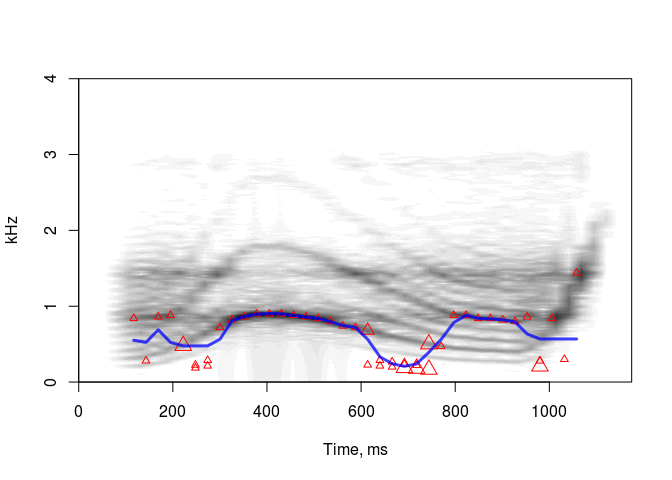TIP As you can guess by now, any pitch tracking method can be tweaked to produce reasonable results for any one particular sound (read: to agree with human intuition). The real trick is to find settings that are accurate on average, across a wide range of sounds and recording conditions. The default settings in `analyze` are the result of optimization against manually verified pitch measurements of a corpus of 260 human non-linguistic vocalizations. For other types of sounds, you will need to perform your own manual tweaking and/or formal optimization.

## 2.5 Postprocessing of pitch contour

Pitch postprocessing in soundgen includes a whole battery of distinct operations through which the pitch candidates generated by one or more tracking methods are integrated into the final pitch contour. We will look at them one by one, in the order in which they are performed in `analyze`. But first of all, here is how to disable them all:

``````a = analyze(
s1,
samplingRate = 16000, plot = TRUE, ylim = c(0, 4), priorMean = NA,
shortestSyl = 0, shortestPause = 0,  # any length of voiced fragments
interpolWin = NULL,     # don't interpolate missing F0 values
pathfinding = 'none',   # don't look for optimal path through candidates
snakeStep = NULL,       # don't run the snake
smooth = NULL           # don't run median smoothing
)       ``````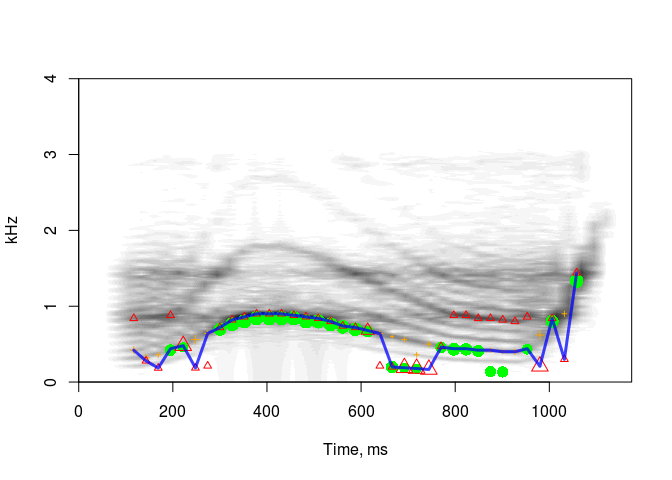When the sound is not too tricky and enough pitch candidates are available, postprocessing actually makes little difference. In terms of the accuracy of median estimate of F0, you are likely to get a good result even with postprocessing is completely disabled. However, if you are interested in the actual intonation contours, not just the global average, postprocessing can help a lot.

### 2.5.1 Continuous voiced fragments

It often makes sense to make assumptions about the possible temporal structure of voiced fragments, such as their minimum expected length (`shortestSyl`) and spacing (`shortestPause`). If these two parameters are positive numbers, the first stage of postprocessing is to divide the sound into continuous voiced fragments that satisfy these assumptions. The default minimum length of a voiced fragment is a single FFT frame. If `shortestSyl` is longer than a single frame, then we need at least two adjacent voiced frames to start a new voiced fragment. A single voiced frame surrounded by unvoiced frames then gets discarded (assumed to be unvoiced). If two voiced fragments are separated by less than `shortestPause`, they are merged. What this means is simply that they are processed as a single syllable by `pathfinder()` (see below). No interpolation takes place at this stage.

The next few blocks of postprocessing are performed by an internal function, `soundgen:::pathfinder()`. Its input is a matrix of pitch candidates for each frame of a single voiced syllable, usually with multiple candidates per frame. Each candidate is also associated with a different certainty. We want to find a good path through these candidates - that is, a pitch contour that both passes close to the strongest candidates and minimizes pitch jumps, producing a relatively smooth contour. The simplest first approximation is to take a mean of all pitch candidates per frame weighted by their certainty - the “center of gravity” of pitch candidates - and for each frame to select the candidate that lies closest to this center of gravity. This initial guess at a reasonable path may or may not be processed further, depending on the settings described below.

### 2.5.2 Interpolation

To make sure we have at least one pitch candidate for every frame in the supposedly continuous voiced fragment, we interpolate to fill in any missing values. The same algorithm also adds new pitch candidates with certainty `interpolCert` if a frame has no pitch candidates within `interpolTol` of the median of the “center of gravity” estimate over plus-minus `interpolWin` frames. The frequency of new candidates is equal to this median. `interpolTol` defaults to 0.05, which means that new candidates are calculated if there are none within 0.95 to 1.05 times the median over the interpolation window. You can also enable interpolation to fill in unvoiced frames, but without adding new pitch candidates in voiced frames. To do so, set `interpolTol = Inf`.

As an illustration, compare the output of the following two commands:

``````par(mfrow = c(1, 2))
a1 = analyze(s1, samplingRate = 16000, plotSpec = FALSE, priorMean = NA,
pitchMethods = 'cep', cepThres = .35, step = 25,
snakeStep = NULL, smooth = 0,
interpolWin = NULL, pathfinding = 'none',  # disable interpolation
main = 'No interpolation')
a2 = analyze(s1, samplingRate = 16000, plotSpec = FALSE, priorMean = NA,
pitchMethods = 'cep', cepThres = .35, step = 25,
snakeStep = NULL, smooth = 0, main = 'Interpolation')

par(mfrow = c(1, 1))``````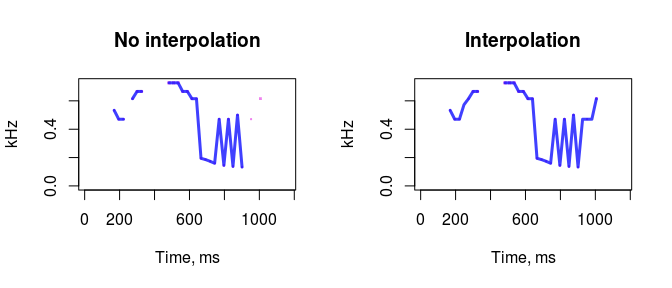TIP: if you turn off interpolation by setting `interpolWin = NULL`, pathfinder may crash if there are unvoiced gaps in the syllable. In practice, you should either disable interpolation and pathfinding together or set `shortestPause = 0` to ensure that each continuous voiced fragment, no matter how short, is treated as a separate syllable

### 2.5.3 Pathfinding

The next step after interpolation is pathfinding proper - searching for the optimal path through pitch candidates. If `pathfinding = "none"`, this step is skipped, so we just continue working with the path that lies as close as possible to the (possibly interpolated) center of gravity of pitch candidates. If `pathfinding = "fast"` (the default option), a simple heuristic is employed, in which we walk down the path twice, first left to right and then right to left, trying to minimize the cost measured as a weighted mean of the distance from the center of gravity and the deviation from a smooth contour. The key setting is `certWeight`, which specifies how much we prioritize the certainty of pitch candidates vs. pitch jumps / the internal tension of the resulting pitch curve. Low `certWeight` (close to 0): we are mostly concerned with avoiding rapid pitch fluctuations in our contour. High `certWeight` (close to 1): we mostly pay attention to our certainty in particular pitch candidates. The example below is intended as an illustration of how pathfinding works, so all other types of smoothing are disabled, forcing the final pitch contour to pass strictly through existing candidates.

``````par(mfrow = c(1, 2))
a1 = analyze(s1, samplingRate = 16000, plotSpec = FALSE, priorMean = NA,
pitchMethods = 'cep', cepThres = .15, nCands = 3,
snakeStep = NULL, smooth = 0, interpolTol = Inf,
certWeight = 0,  # minimize pitch jumps
main = 'Minimize jumps')
a2 = analyze(s1, samplingRate = 16000, plotSpec = FALSE, priorMean = NA,
pitchMethods = 'cep', cepThres = .15, nCands = 3,
snakeStep = NULL, smooth = 0, interpolTol = Inf,
certWeight = 1,  # minimize deviation from high-certainty candidates
main = 'Pass through candidates')
par(mfrow = c(1, 1))``````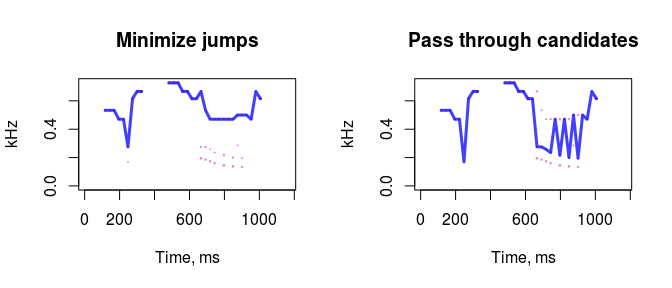The final option is `pathfinding = 'slow'`, which calls `stats::optim(method = 'SANN')` to perform simulated annealing. This is a more powerful algorithm than the simple heuristic in `pathfinding = 'fast'`, but it is called “slow” for a good reason. In case you have plenty of time, it does improve the results, but note that this algorithm is stochastic, so each run may produce different results. Use an additional argument, `annealPars`, to control the algorithm. See `?stats::optim` for more details.

### 2.5.4 Snake

What is here esoterically referred to as the “snake” can be seen as an alternative to the pathfinding algorithms above, although both can also be performed sequentially. Whereas pathfinding attempts to find the best path through existing pitch candidates, the snake wiggles the contour under a weighted combination of (a) elastic forces trying to snap the pitch contour to a straight line and (b) the pull of high-certainty pitch candidates. In a sense the snake is thus a combination of interpolation and pathfinding: like interpolation, it can add new values different from existing candidates, and like pathfinding, it balances the certainty in candidates against the smoothness of the resulting contour.

The only new control parameter in the snake module (apart from `certWeight`) is `snakeStep`, which controls the speed of adaptation (the default is 0.05). The higher it is, the faster the snake “wiggles”. This reduces processing time, but introduces a risk of “overshooting”. If `snakeStep` is too low (close to 0), the snake moves too slowly and may fail to reach its optimal configuration. To disable the snake module, set `snakeStep = NULL`. You can also produce a separate plot of the snake by setting `snakePlot = TRUE`, as in the example below (again, all other postprocessing is disabled to show what the snake alone will do). The zigzagging line is the initial contour (the path through pitch candidates that lie as close as possible to the center of gravity of each frame), the smooth blue line is the pitch contour after running the snake, and the green lines trace the progress of iterative snake adaptation. Note that at `certWeight = 0.1` the snake is heavily biased towards producing a smooth contour, regardless of its distance from high-certainty pitch candidates.

``````a1 = analyze(s1, samplingRate = 16000, plot = FALSE, priorMean = NA,
pitchMethods = 'cep', cepThres = .2, nCands = 2,
pathfinding = 'none', smooth = 0, interpolTol = Inf,
certWeight = 0.1,  # like pathfinding, the snake is affected by certWeight
snakeStep = 0.05, snakePlot = TRUE)``````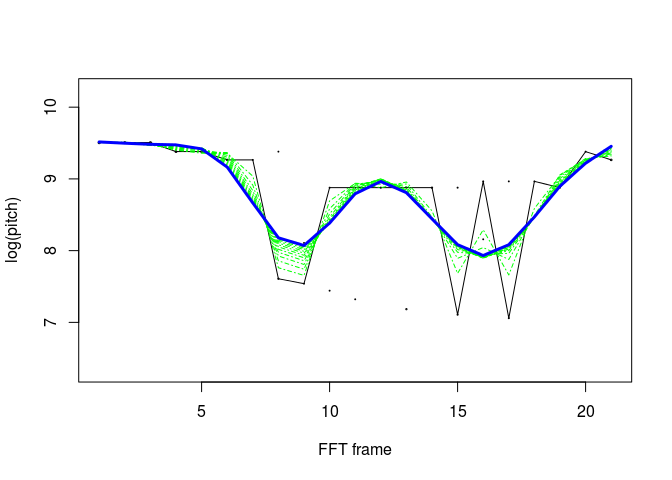TIP Should you use pathfinding, the snake, or both? Pathfinding makes more sense if you want the final contour to pass strictly through existing candidates, say if there are relatively few candidates, most of which are right on target and some completely off. In these conditions the snake will not do much (but not much harm, either). The snake becomes attractive if you have a lot of candidates from different pitch tracking methods, many of which are slightly off and should be averaged. In addition, the more garbage you expect among your pitch candidates, the more you might want to extrapolate and apply median smoothing

### 2.5.5 Median smoothing

The final postprocessing stage is median smoothing. It is conceptually similar to interpolation, except that by now there is only a single F0 value left per frame, so we can forget about the multiple candidates and their certainties. It wouldn’t make much sense to apply kernel smoothing to this curve: the snake can usually do this in a smarter way, since it does know about the multiple candidates and their certainties. What we want from the smoothing algorithm is to detect and correct only outliers: the values that stick out from the surrounding frames. The parameters that control this module are `smooth` and `smoothVars`.

If `smooth` is a positive number, contours of the variables in `smoothVars` are smoothed using a customized version of median smoothing. This modifies only the values that deviate considerably from the moving median and preserves all other values (so this is a bit different from applying a moving median or kernel smoothing). `smooth` controls both the tolerated deviance and the size of the window for calculating a moving median. `smooth = 1` (the default) corresponds to a window of ~100 ms and tolerated deviation of ~4 semitones. This smoothing can be applied to any measured value, not only the final pitch contour. The default is `smoothVars = c('pitch', 'dom')`. To turn off the median smoothing, set `smooth = NULL` or `smoothVars = NULL`.

``````par(mfrow = c(1, 2))
a1 = analyze(s1, samplingRate = 16000, plotSpec = FALSE, priorMean = NA,
pitchMethods = 'cep', cepThres = .2, nCands = 2,
pathfinding = 'none', snakeStep = NULL, interpolTol = Inf,
smooth = 0, main = 'No smoothing')
a2 = analyze(s1, samplingRate = 16000, plotSpec = FALSE, priorMean = NA,
pitchMethods = 'cep', cepThres = .2, nCands = 2,
pathfinding = 'none', snakeStep = NULL, interpolTol = Inf,
smooth = 1, main = 'Default smoothing')
par(mfrow = c(1, 1))``````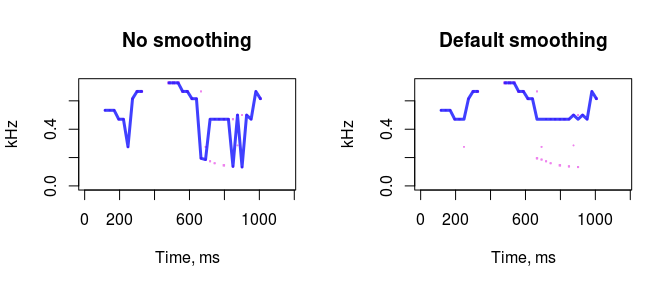TIP Pathfinding (“slow”, “fast” or “none”) is the only postprocessing module that does not deviate from pitch candidates actually returned by pitch tracking algorithms. Interpolation, snake, and median smoothing produce new pitch values per frame, which may be quite different from any actual candidates

## 2.6 Customization of pitch plotting

When analyzing a sound, and even when batch-processing an entire folder, it is often helpful to plot both the final pitch contour - perhaps overlaid on a spectrogram - and individual pitch candidates. You can easily do so from `analyze`, as in all the examples above. The default plotting parameters can also be customized, for example:

``````a = analyze(s1, samplingRate = 16000, plot = TRUE, priorMean = NA,
# options for spectrogram(): see ?spectrogram
contrast = .75,
brightness = -0.5,
colorTheme = 'seewave',
ylim = c(0, 4),
# + other pars passed to seewave::filled.contour.modif2()

# options for plotting the final pitch contour (line)
pitchPlot = list(
col = 'black',
lwd = 5,
lty = 3
# + other pars passed to base::lines()
),

# options for plotting pitch candidates (points)
candPlot = list(
levels = c('autocor', 'cep', 'spec', 'dom'),
col = c('green', 'violet', 'red', 'orange'),
pch = c(16, 7, 2, 3),
cex = 3
))``````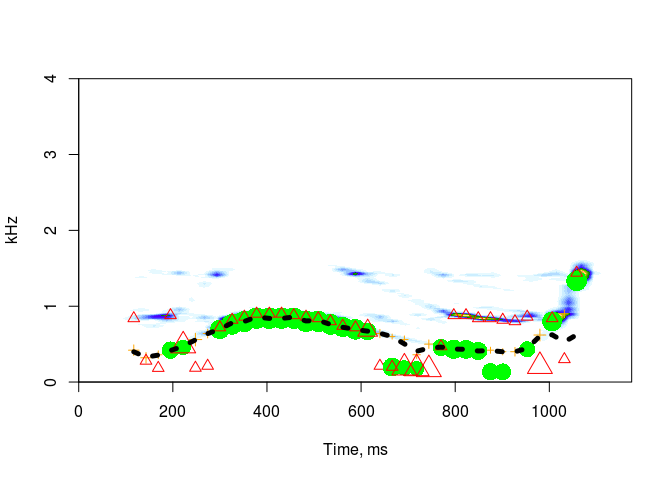You can also suppress plotting any of these three components: the spectrogram, the final pitch contour, or individual pitch candidates. To suppress plotting the spectrogram, set `plotSpec = FALSE`. To suppress plotting the pitch contour, set `pitchPlot = NULL`. To suppress plotting pitch candidates, set `candPlot = NULL`.

To save the plot, specify a valid path, for example:

``````a = analyze(s1, samplingRate = 16000, plot = TRUE, savePath = '~/Downloads',
width = 900, height = 500, units = 'px')``````

This creates a file called ‘sound.jpg’ 900 x 500 pixels in size in the indicated folder. This is mostly useful when you do batch processing of multiple files with `analyzeFolder` and want to save the plots for manual checking of extracted plot contours (see below).

## 2.7 Batch processing with `analyzeFolder`

You may not feel too excited to learn that soundgen contains a wrapper around `analyze` that is meant for analyzing all .wav files in a folder. Indeed, calling `analyze` in a loop will achieve the same result. However, `analyzedFolder` can save you a bit of manual coding. If you want to preserve frame-by-frame information for each file in a folder, you can either loop through the files manually or call `analyzeFolder(myfolder, summary = FALSE)`, which returns a list of dataframes. In contrast, `analyzeFolder(myfolder, summary = TRUE)` returns a single dataframe, in which each acoustic predictor is summarized as the mean, median, and SD of frame-by-frame measurements. Since this is the kind of data you would normally use as input for things like classification of sounds or cluster analysis, this is a convenient shortcut for generating an acoustic dataset for further statistical modeling. In addition, `analyzeFolder` allows you to simultaneously save the plots and prints out estimated time to completion.

TIP Processing time varies a lot depending on the exact settings and input, but expect up to a few seconds of machine time per second of audio. The surest way to speed things up is to reduce `step` and to avoid `pathfinding = 'slow'` (other types of postprocessing have very little effect on processing time)

# 3 Audio segmentation

## 3.1 Bursts and syllables

### 3.1.1 Segmenting a single sound: `segment`

In addition to measuring spectral characteristics and fundamental frequency, it is often important to analyze the temporal structure of a sound. In particular, it is often helpful to divide a sound into separate “syllables” - continuous acoustic fragments separated by what we consider to be “silence”. If this “silence” was not full of background noise and breathing, the task would be trivial: we could simply define syllables as continuous segments with ampiltude above some threshold. As it is, with non-studio material it is problematic to find a single threshold that would accurately coincide with the beginning and end of syllables in different sounds (presuming that we are interested in batch processing multiple sounds without manually adjusting the settings for each sound).

Sometimes we are more interested in the rate of syllables per second and in their regularity, rather than the absolute duration of each syllable. In this case it makes more sense to look for bursts of acoustic energy - local maxima in amplitude envelope that are high enough both in absolute terms (relative to the global maximum) and with respect to the surrounding region (relative to local mimima). The spacing between bursts - the interburst interval - can allow us to recover the perceptually salient temporal structure of a bout of vocalizing, such as the number of syllables in a bout of laughing, their average frequency, and regularity.

Soundgen package contains a function called `segment`, which uses both these approaches: it looks for both syllables and bursts. These two algorithms are not independent: syllables are found first, and then the median length of a syllable becomes the expected interburst interval, guiding burst detection. `segment` operates with amplitude envelopes - smoothed contours of sound intensity. To demonstrate how it works, we will look at the example from `?segment` and go through the control parameters.

``````# for info on using soundgen() function, see the vignette on sound synthesis
s2 = soundgen(nSyl = 8, sylLen = 50, pauseLen = 70, temperature = 0,
pitch = c(368, 284),
noise = list(time = c(0, 67, 86, 186),
value = c(-45, -47, -89, -120)),
rolloffNoise = -8, amplGlobal = c(0, -20))
# spectrogram(s2, samplingRate = 16000, osc = TRUE)
# playme(s2, samplingRate = 16000)
a = segment(s2, samplingRate = 16000, plot = TRUE)``````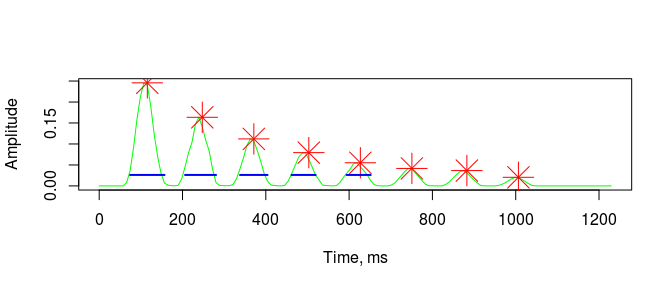This synthesized laugh-like sound contains 8 syllables, each 50 ms long and separated by 70 ms of unvoiced fragments with some overlapping aspiration noise (NOT silence!). With default settings, `segment` finds 5 syllables of median length `median(a\$syllables\$sylLen, na.rm = TRUE)` = 66 ms separated by `median(a\$syllables\$pauseLen, na.rm = TRUE)` = 57 ms. The syllables are shown by blue line segments in the plot above. The last syllables are missed either because they are below the default detection threshold `sylThres` (90% of the global mean amplitude) or because their apparent duration falls below the default `shortestSyl` of 40 ms due to its low amplitude. All 8 bursts are correctly detected. The interburst interval is estimated to be `median(a\$bursts\$interburstInt, na.rm = TRUE)` = 123 ms (the correct number is 120 ms), with SD = `sd(a\$bursts\$interburstInt, na.rm = TRUE)` = 4 ms (the correct number is 0, since we set temperature to 0). Note that, just as with many real-life sounds, the question of when each syllable starts and ends is pretty meaningless, given the continuous and loud breathing noise. In contrast, the spacing of bursts is both perceptually meaningful and objectively measurable.

Some other settings worth mentioning are:

• `windowLength`, `overlap`: length (ms) and overlap (%) of the smoothing window used to produce the amplitude envelope. See `?seewave::env`, which is called with the argument msmooth = c(smooth_points, smoothOverlap), where smooth_points = ceiling(windowLength * samplingRate / 1000). Setting the overlap too low makes the enveloped jagged and imprecise, while setting the window length too low produces insufficient smoothing:
``````par(mfrow = c(3, 1))
a1 = segment(s2, samplingRate = 16000, plot = TRUE,
windowLength = 40, overlap = 0)   # overlap too low
a2 = suppressWarnings(segment(s2, samplingRate = 16000, plot = TRUE,
windowLength = 5, overlap = 80))  # window too short
a3 = segment(s2, samplingRate = 16000, plot = TRUE,
windowLength = 150, overlap = 80) # window too long
par(mfrow = c(1, 1))``````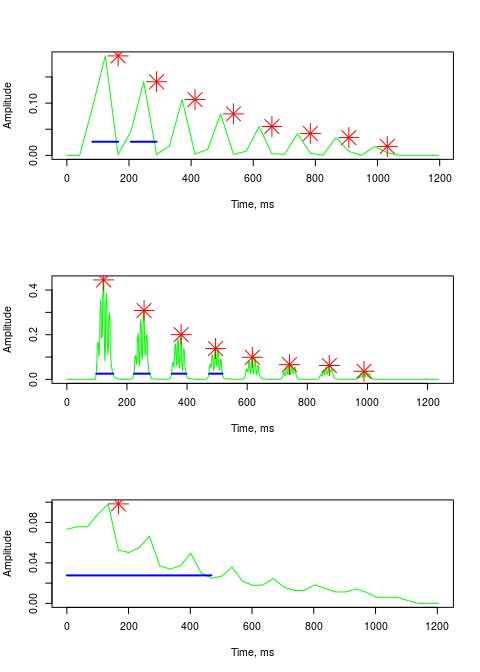• `shortestSyl` and `shortestPause` incorporate our prior knowledge by expecting the syllables and pauses between them to be at least 40 ms long (by default). Setting `shortestSyl` and `shortestPause` too low may inflate the number of discovered syllables, but burst detection should not be affected as much. If `shortestSyl` is too high, excluding shorter fragments, we won’t find any syllables, but burst detection can still succeed. The most damaging mistake is to set `shortestPause` too high, because separate syllables are then merged and the expected interburst interval becomes inflated, preventing the algorithm from recognizing closely spaced bursts (see the example below).
``````par(mfrow = c(2, 1))
a1 = segment(s2, samplingRate = 16000, plot = TRUE,
shortestSyl = 80)    # too long, but at least bursts are detected
a2 = segment(s2, samplingRate = 16000, plot = TRUE,
shortestPause = 80)  # merges syllables
par(mfrow = c(1, 1))``````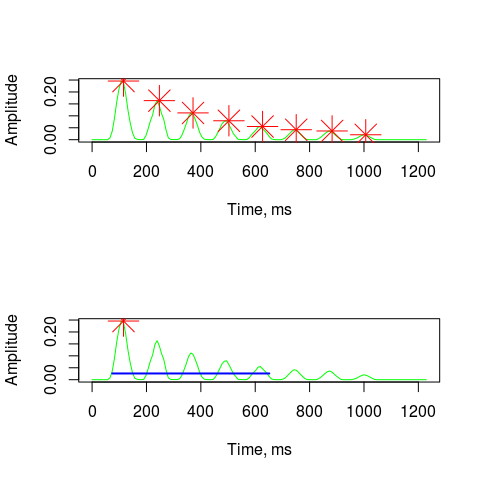• `burstThres` and `peakToTrough` control how high a burst has to be in absolute terms (compared to the global maximum of the envelope) and in relative terms (compared to the local minimum). So a burst is a local maximum that is at least `burstThres` high and at least `peakToTrough` times higher than the local minimum. The size of the analysis window for finding local maxima and minima is controlled by `interburst`, which defaults to the median length of detected syllables times `interburstMult`. By default the local minimum is only calculated to the left of the candidate burst (`troughLeft = TRUE, troughRight = FALSE`). The left-right distinction is irrelevant with this artificial, perfectly symmetrical example, but it appears to improve performance with real-life sounds, which often display assymmetrical attack with sharp onset and more gentle decay.
``````par(mfrow = c(2, 1))
# absolute threshold burstThres set too high
a1 = segment(s2, samplingRate = 16000, plot = TRUE,
burstThres = 0.5)
# improper syllable merging due to shortestPause, but overriden by manually
# specified interburst
a2 = segment(s2, samplingRate = 16000, plot = TRUE,
shortestPause = 80, interburst = 100)
par(mfrow = c(1, 1))``````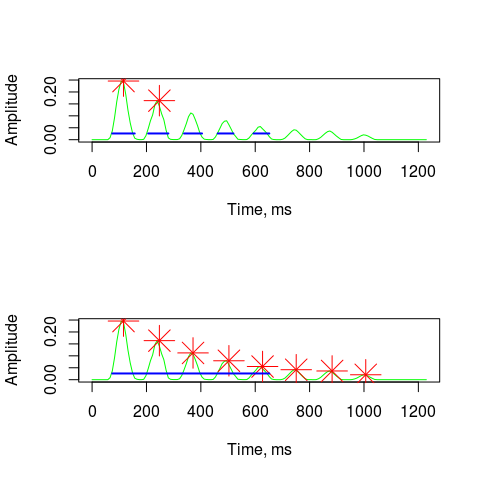### 3.1.2 Batch processing: `segmentFolder`

Just as `analyzeFolder` is a wrapper around `analyze` that simplifies looping through a whole folder of .wav files, `segmentFolder` provides a convenient wrapper around `segment`. It accepts the same arguments as `segment` and can optionally save segmentation plots in the designated folder (e.g., `savePath = ~/Downloads/`). It also reports estimated time left (`verbose = TRUE`). You can save either detailed stats on individual syllables and bursts (`summary = FALSE`) or produce a summary table with means, medians, and SDs (`summary = TRUE`).

TIP If you are analyzing a single sound and are willing to adjust the settings manually, you can measure both syllables and bursts accurately. For batch processing without manual adjustments, bursts are more robust

## 3.2 Self-similarity matrices

The basic idea behind self-similarity matrices (SSMs) is to compare different parts of the same sound with each other and present their pairwise similarity indices as a square matrix with time along both dimensions. The diagonal going from bottom-left to top-right represents the similarity of each fragment with itself. For example, at (100, 100) we have the similarity of the fragment at 100 ms with itself, while at (100, 200) we have the similarity of the fragments at 100 ms and 200 ms. Let’s begin with an example taken from `?ssm`:

``````s3 = c(soundgen(), soundgen(nSyl = 4, sylLen = 50, pauseLen = 70,
formants = NA, pitch = c(500, 330)))
# playme(s3, 16000)
m = ssm(s3, samplingRate = 16000)``````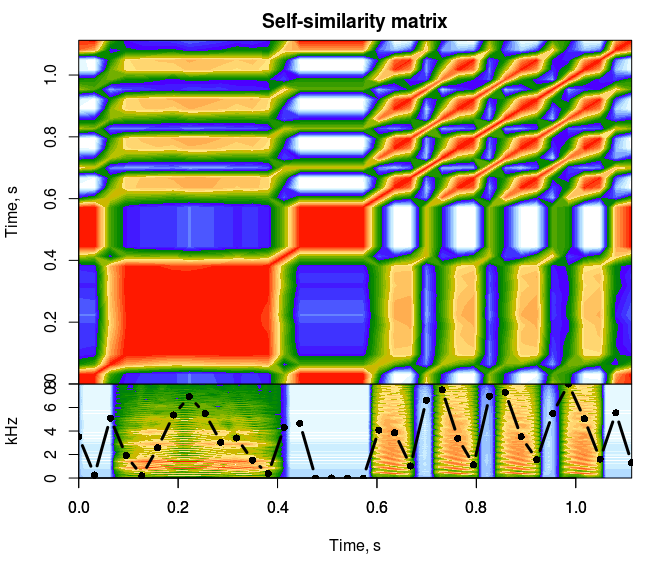Look at the upper panel first. There are three main regions: the large red square in the lower left corner, which corresponds to the first bout; the red square in the center, which corresponds to the long pause between the bouts; and the checkered field in the upper right corner, which corresponds to the second bout. Observe the characteristic diagonal. The SSM clearly shows periodicity within the second bout, which confirms that these syllables are very similar to each other in terms of their spectral characteristics. Now look at the lower panel of the plot. This is a spectrogram overlaid with so-called “novelty”: peaks show when something changes in the sound, based on the SSM.

The results of SSM analysis can vary dramatically, depending on how it is performed. We begin by extracting some form of spectrogram of the entire sound, then we somehow compare each frame to each other frame, and finally we calculate a measure of novelty. At each step there are crucial decisions to make, which can dramatically alter the result. Let’s look at the settings that control each step. As you play with these settings, don’t be surprised if your SSM suddenly looks completely different!

1. Spectrogram extraction is controlled by some already familiar settings such as `windowLength` and `overlap` (or `step` if you prefer). However, it can often be helpful to base the SSM on some other spectral characteristics than the usual spectrogram. Under the hood, `ssm` calls `tuneR::melfcc` to return the power spectrum (as in a spectrogram, `input = 'spectrum'`), mel-transformed spectrum (auditory spectrum, which is supposed to be closer to how humans perceive sounds, `input = 'audiogram'`), or mel-frequency cepstral coefficients (MFCCs - a popular choice of input for automatic sound classification, as in speech recognition software, `input = 'mfcc'`). There are also several arguments that are passed to `tuneR::melfcc`: `maxFreq` controls the highest frequency analyzed, `MFCC` controls the number of mel-frequency cepstral coefficients to extract, and `nBands` basically controls the frequency resolution.

2. SSM calculation starts with the spectrogram (or the MFCCs matrix) produced at the previous stage. This input is divided into windows, each of which can be one or several FFT frames in length, and then each window is compared to each other window using either cosine similarity (`simil = 'cosine'`) or Pearson’s correlation (`simil = 'cor'`). Prior to this the input matrix can be normalized column-wise, so as to level out the differences in sound intensity across frames (`norm = TRUE`). The size of analysis window is controlled by the argument `ssmWin`, ms. Note that as you increase `ssmWin`, the resulting SSM literally shrinks in size, reducing its resolution. This is a great feature if your sound is very long, but it is seldom needed for shorter sounds. As you decrease `ssmWin`, it ultimately reaches the size of a single FFT frame (`step`) and can’t go down any further, unless you improve time resolution of the spectrogram itself by reducing `windowLength` and/or increasing `overlap`.

3. Novelty calculation starts with the SSM computed at the previous step. Here the idea is to move along the diagonal and multiply the SSM by a Gaussian checkerboard matrix, so as to detect changes in the spectral structure (see Foote, 2000). The settings that control this algorithm are `kernelLen` and `kernelSD`. The crucial parameter is `kernelLen` (ms): a large kernel is good for detecting global changes in the sound, such as transitions between different vocalizations, whereas a small kernel is good for detecting rapid changes, such as individual bursts in a laugh. If you are curious about the kernel itself, take a look at `?soundgen:::getCheckerboardKernel()`.

``````par(mfrow = c(2, 1))
m1 = ssm(s3, samplingRate = 16000,
input = 'audiogram', simil = 'cor', norm = FALSE,
ssmWin = 10, kernelLen = 150)  # detailed, local features
m2 = ssm(s3, samplingRate = 16000,
input = 'mfcc', simil = 'cosine', norm = TRUE,
ssmWin = 50, kernelLen = 600)  # more global
par(mfrow = c(1, 1))``````